# Dielectric Spectroscopy and Impedance Spectroscopy of Materials

The aim here is to characterize the electric or magnetic properties of materials by the impedance spectrum Z*(ω). To this end, the material is placed into a sample cell. Electrical characterization is performed by measuring the complex permittivity spectra ε*(ω) and conductivity spectra σ*(ω). These are related to the dynamics of charge transport and dipole reorientation in the material.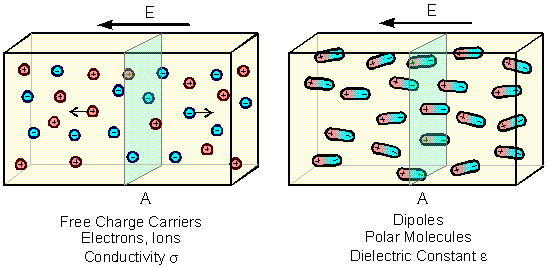Usually, materials contain both free charge carriers (electrons, ions) and charge carriers bound in molecules. If the electrical properties are goverend by the free charge carriers, they are classfied as conductors, otherwise as dielectric materials. If an electric field E is applied to a material, the free charge carriers will move through it, and the polar molecules will partly be oriented in the direction of the field. This is described by the current density js or the polarization Ps.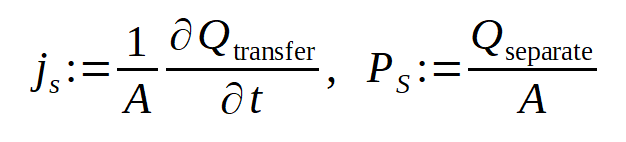For the free charge carriers, A is the area where the charge dQtransfer flows through within the time dt. For the molecules A is the area which separates the amount of charge Qseparate due to the oriented dipoles.

After a sufficient time, the current density and the polarization will become constant in time, and the material will be in a static state. In this case the static material constants conductivity σs and permittivity εs can be defined by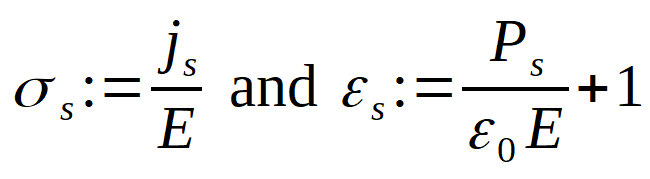They describe the current density or polarization response of a material with respect to the electric field.

After the electric field is applied to the material, the free charge carriers will not imediately reach their final speed and the molecules will need a certain time for orientation. As a result all quantities defined above will become time dependent.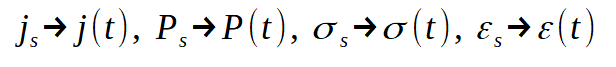This time dependence is called charge or molecular relaxation. It can be directly measured by time domain spectroscopy methods, where the flow of charge is measured as a function of time after switching on the electric field.

In most cases, however, data are measured and evaluated in the frequency domain, which offers three major advantages.
• Data can be measured more accurately and over a much larger conductance and permittivity range.
• Relaxation phenomena are decribed in the time domain by linear differential equations, whereas the corresponding frequency representation contains simpler algebraric terms with powerlaws in frequency. The frequency and time domain representations in principle contain the same information and are linked by Fourier Transforms.
• Data directly link up with other spectrocopy techniques in the optical and UV range.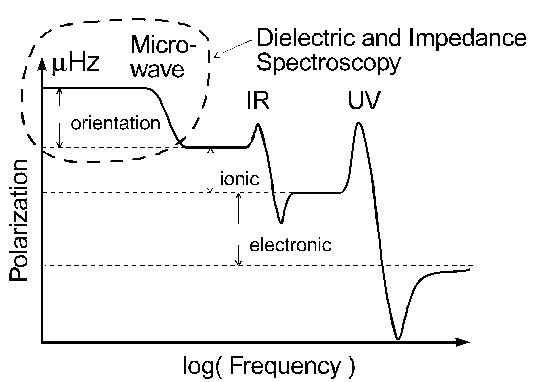Fig. Typical frequency range of charge relaxation and resonance effects.

In a frequency domain measurement instead of a step, an electrical field oscillating with a fixed frequency ω/(2π) is applied to the material. The materials response is an oscillating charge at the same frequency. If in eqns. (x-y) the static field is replaced by an oscillating field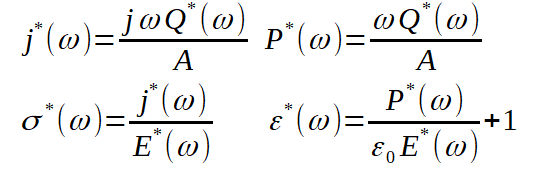(The current density j*(ω) should not be confused with imaginary unit j). Both in a frequency domain and in the time domain measurement, the problem arises that both the dipoles and the free charge carriers will contribute time or frequency dependent charge parts.

In the equations above, the free charge response Q*transfer(ω) is 90° shifted with respect to the driving field, whereas the dipole charge response Q*separate(ω) is in phase with the field. Therefore, in principle, the both contributions can be separated by phase. In pratice, this works only if the frequency is sufficiently low, so that dynamic effects of the free charge or dipole response related to their mass can be neglected. The materials electrical response is than frequency independent and Re(ε*(ω)) = εs, Re(σ*(ω)) = σs (static case).

If dynamic effects become relevant, dipole relaxation is always damped resulting in a non zero phase shift of Q*separate(ω) against E*(ω). In addition, the free charge carrier response often shows a deviation from the ideal 90° phase shift of Q*transfer against E*(ω). This may become relevant in the frequency range of dielectric or impedance spectroscopy especially for ions with a large mass and for molecular dipoles.

In a single frequency measurement, generally the two contributions can not be separated from each other and sum up to the totally measured charge oscillation

Q*(ω) = Q*transfer(ω) + Q*separate(ω)

used in the eqns above. With this, both ε*(ω) and σ*(ω) (and j*(ω) and P*(ω)) are refered to the total charge and contain the same information inlcuding free charge carrier and dipole response information. In this sense, ε*(ω) and σ*(ω) can be seen as different representations for the materials charge response to an electric field.

For pure conductive materials like metals or pure dielectrics like Silica, the relaxation times are fast and outside the measurement range of dielectric or impedance spectroscopy. In a lot of other materials, however, the movement of charge or molecules is hindered by, e.g., the materials vicosity. In these cases, the relaxation times are often in the range from some 10 ps to weeks. This corresponds to frequencies from m seconds to some GHz and can be measured by dielectric or impedance spectroscopy. It applies especially for polymer materials where the molecular dynamics is hindered by the entanglement of the molecular chains.